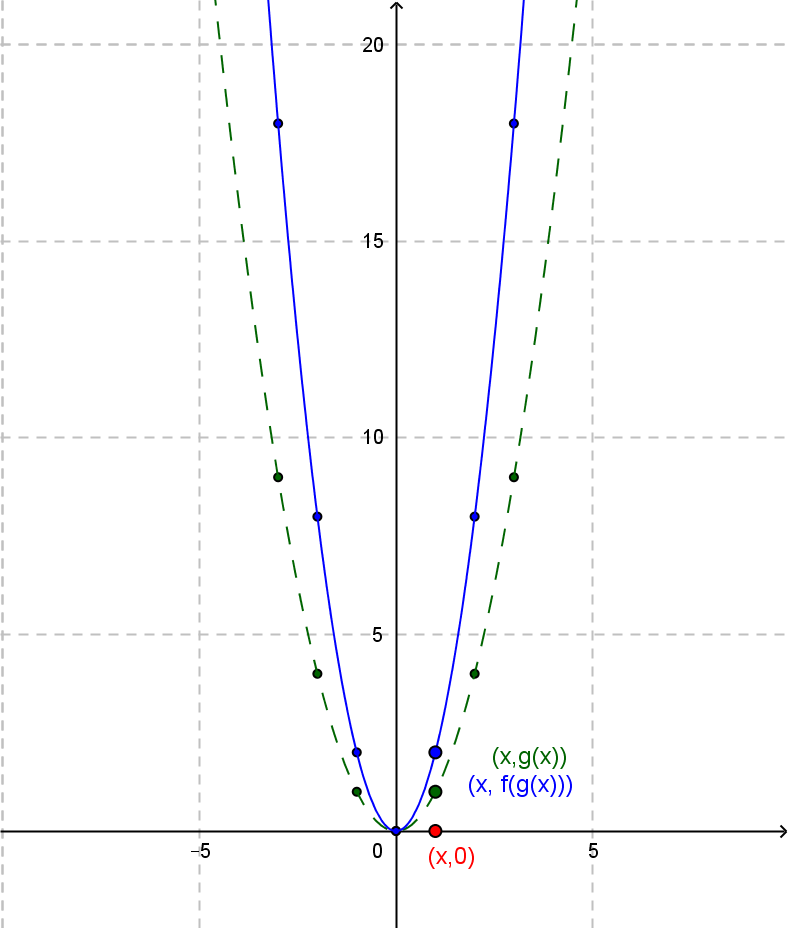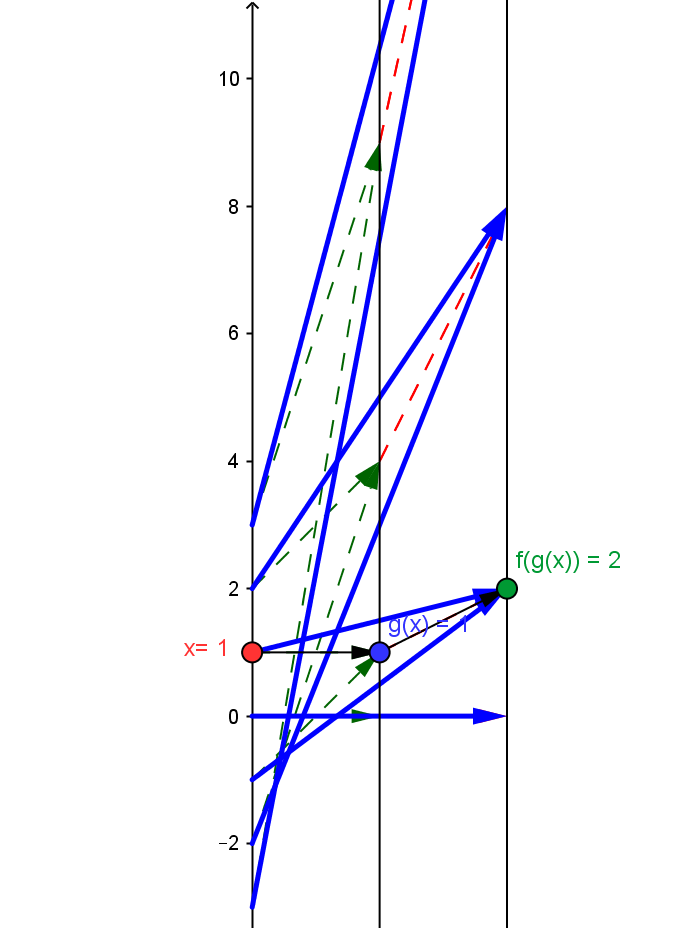Example QF.2.2 : \$A =2; q(x) = 2x^2\$
This is best understood as the composition of the core quadratic function \$g(x)= x^2\$ followed by the linear function \$f(x) = 2x\$, so \$q(x) = f(g(x))\$.
Draw a mapping diagram yourself or  use the diagram created with GeoGebra to explore the diagram further.
Compare the mapping diagram with the graphs of \$g(x)\$ and \$q(x)\$
 Graphs of \$g(x)\$ and \$q(x)\$Mapping Diagram Showing Composition. Given a point / number, \$x\$, on the source line, there is a blue arrow meeting the target line at the point / number, \$2x^2\$. This point corresponds to the quadratic function's value for \$x\$. The values for the core mapping diagram for \$x^2\$ in green are magnified by a factor of \$2\$  shown by the red arrows.As \$x\$ increases, \$q(x)\$ decreases to value \$q(0)=0\$ and then increases.